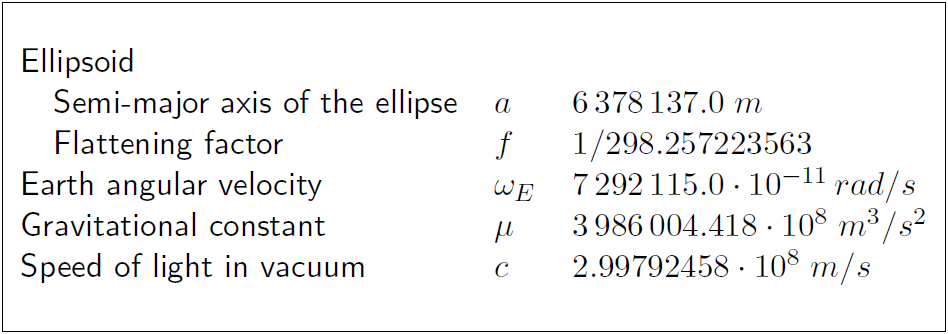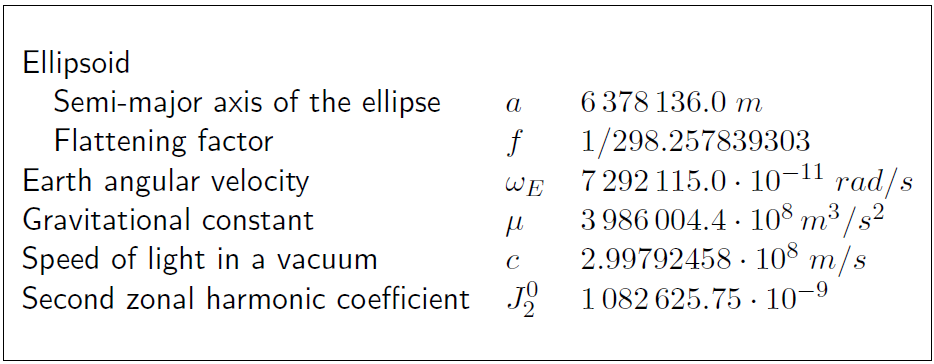If you wish to contribute or participate in the discussions about articles you are invited to join Navipedia as a registered user

# Reference Frames in GNSSFundamentals
Title Reference Frames in GNSS
Author(s) J. Sanz Subirana, J.M. Juan Zornoza and M. Hernández-Pajares, Technical University of Catalonia, Spain.
Level Basic
Year of Publication 2011

Brief descriptions of GPS WGS-84, GLONASS PZ-90 and Galileo GTRF reference frames are provided as follows.

## GPS reference frame WGS-84

From 1987, GPS uses the World Geodetic System WGS-84, developed by the US Department of Defense (DoD), which is a unified terrestrial reference system for position and vector referencing [footnotes 1]. Indeed, the GPS broadcast ephemeris are linked to the position of the satellite antenna phase centre in the WGS-84 reference frame. Thus, the user receiver coordinates will be expressed in the same ECEF frame.

The initial implementation of WGS-84 was realized from a set of more than a thousand terrestrial sites, which coordinates were derived from Transit observations [footnotes 2]. Successive refinements (which also leaded to some adjustments of the fundamental constants), using more accurate coordinates of the monitor stations, approximate to some ITRS realizations. For instance, realizations WGS84(G730) [footnotes 3] and WGS84(G873) correspond to ITRF92 and ITRF94, respectively. The refined frame WGS84(G1150) was introduced in 2002, which agrees with ITRF2000 at the centimetre level.

The parameters of the WGS-84 ellipsoid are given in the following table 1:Table 1: Ellipsoidal parameters WGS-84 (revised in 1997).

## GLONASS reference frame PZ-90

The GLONASS broadcast ephemeris are given in the Parametry Zemli 1990 (Parameters of the Earth 1990) (PZ-90) reference frame. As the WGS-84, this is an ECEF frame with a set of fundamental parameters associated (see table 2 from [GLONASS ICD, 2008]).

The determination of a set of parameters to transform the PZ-90 coordinates to the ITRF97 was the target of the International GLONASS Experiment (IGEX-98). [Boucher and Altamimi, 2001] presents a review of the IGEX-98 experiment and, as a conclusion, they suggest the following transformation [footnotes 4] from $(x,y,z)$ in PZ-90 to $(x',y',z')$ in WGS-84, with a meter level of accuracy.

$\left [ \begin{array}{c} x'\\ y'\\ z'\\ \end{array} \right ] = \left [ \begin{array}{c} x\\ y\\ z\\ \end{array} \right ] + \left [ \begin{array}{ccc} -3\,ppb & -353\,mas & -4\,mas\\ 353\,mas & -3\,ppb & 19\,mas\\ 4\,mas & -19\,mas & -3\,ppb\\ \end{array} \right ] \left [ \begin{array}{c} x\\ y\\ z\\ \end{array} \right ] + \left [ \begin{array}{c} 0.07\,m\\ -0.0\,m\\ -0.77\,m\\ \end{array} \right ] \qquad\mbox{(1)}$

Following the notation of equation (3) in Transformation between Terrestrial Frames:

$\left ( \begin{array}{c} x\\ y\\ z\\ \end{array} \right )_{_{TRF2}} = \left ( \begin{array}{c} x\\ y\\ z\\ \end{array} \right )_{_{TRF1}} + \left ( \begin{array}{c} T_1\\ T_2\\ T_3\\ \end{array} \right ) + \left ( \begin{array}{ccc} D & -R_3 & R_2\\ R_3 & D & -R_1\\ -R_2 & R_1 & D\\ \end{array} \right ) \left ( \begin{array}{c} x\\ y\\ z\\ \end{array} \right )_{_{TRF1}}$

the previous transformation (1) is defined by the parameters table:

According to the GLONASS modernisation plan, the ephemeris information implementing the PZ-90.11 reference system was updated on all operational GLONASS satellites starting from 3:00 pm on December 31, 2013. From this time on, the satellites are broadcasting in the PZ-90.11. This ECEF reference frame is an updated version of PZ-90, closest to the ITRF2000.

The transformation from PZ-90.11 to ITRF2008 contains only an origin shift vector, and no rotations nor scale factor, as it is shown in equation (2) [Revnivykh, 2007]

$\left [ \begin{array}{c} x\\ y\\ z\\ \end{array} \right ]_{ITRF2008} = \left [ \begin{array}{c} x\\ y\\ z\\ \end{array} \right ]_{PZ-90.11} + \left [ \begin{array}{r} 0.003\,m\,\pm0.002\,m\\ 0.001 \,m\,\pm0.002\,m\\ 0.001 \,m\,\pm0.002\,m\\ \end{array} \right ] \qquad\mbox{(2)}$

The parameters associated to the PZ-90 and PZ-90.11 are given in the next table 2 ([GLONASS ICD, 1998] and [GLONASS ICD, 2008]):Table 2: Ellipsoidal parameters of PZ-90 and PZ-90.11.

## Galileo reference frame (GTRF)

A consortium named the Galileo Geodetic Service Provider (GGSP), consisting of seven institutions under the lead of GeoForschungsZentrum Potsdam, built up a prototype for the development of the Galileo Terrestrial Reference Frame (GTRF) and the establishment of a service with products and information for the potential users.

Like GPS, Galileo established a dedicated terrestrial reference frame (GTRF) as an independent realization of the international terrestrial reference system ITRS based on the estimated coordinates for each one of the Galileo Sensor Station (GSS) sites. According to Galileo requirements the three-dimensional differences of the position compared to the most recent ITRF should not exceed 3 cm (2-sigma).

The realization of GTRF is the task of the Galileo Geodetic Reference Service Provider (GRSP). Operational GTRF includes all Galileo sensor stations (GSS) and selected stations of IGS. IGS stations are used for alignment to ITRF (as GSS are not a part of it) and for densification of the network to improve the accuracy of the results.

GTRF computation includes two parts: free network adjustment including all stations (GSS and selected IGS) and network alignment to ITRF using the IGS stations.

GRSP prototype was developed by a consortium of leading European geodetic institutions under an EC 6th framework contract (http://www.ggsp.eu). It established the four first realizations of GTRF:

The network included 131 IGS stations and 13 GESS (Galileo Experimental Sensor Stations for the GIOVE mission) (source: http://www.ggsp.eu)

Already the initial realization of the GTRF called GTRF07v01 was in agreement with ITRF05 up to 0.9, 0.9 and 2.7 mm for North, East, and Up respectively.

The anticipated configuration of the operational GTRF network is presented in the figure below (source: http://www.ggsp.eu).

GTRF transformation parameters to ITRF2005 are reported below (source: http://www.ggsp.eu).

Solution GTRF08v01, epoch 07:241, used number of ITRF2005 sites: 103

Further details on the implementation of the Galileo Terrestrial Reference Frame can be found in the Galileo Open Service - Service Definition Document (SDD)  .